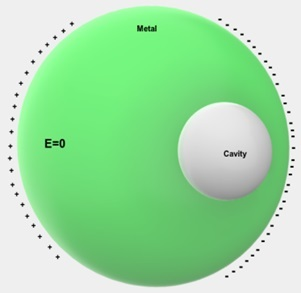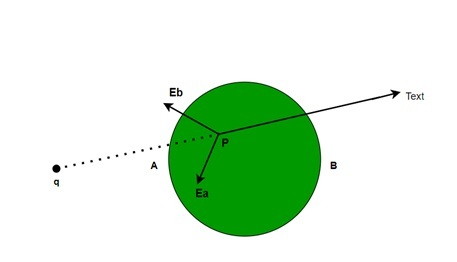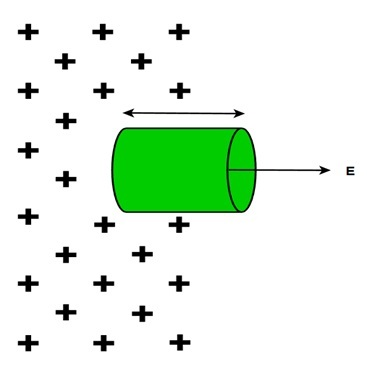# Conductors in Electrostatic Equilibrium

## Introduction

Conductors in electrostatic equilibrium places a major role in shielding objects electrostatically. The movement of protons, electrons, as well as other subatomic particles within material results in the transfer of electric charge from one place to the next, thereby creating an electric current that flows through the substance. The interaction that is produced by the electrostatic forces between the particles facilitates the charges to move around inside materials easily. Thus, Conductors are substances that allow flow of charge with a minimum resistance i. e. substances with free electrons that are not bonded to the atoms.

## What is Electrostatic Equilibrium?

We all know that equilibrium means to be stabilise or have no change. Hence, the term "electrostatic equilibrium" refers to the situation where the charge distribution on a wire is so balanced that the electric field within the wire disappears or we can say a conductor is not experiencing any net charge movement.

## Properties of the Conductor in Electrostatic Equilibrium.

A conductor exhibits the following qualities when it is in electrostatic equilibrium.

• In electrostatic equilibrium, the conductor has no internal electric field. The internal electrical field of the conductor will always be zero, regardless of its shape.

• An isolated conductor only has an electric charge on the surface since it is not in close contact with any other conductors.

• The immediate electric field of a charged conductor coincides perpendicularly with the conductor surface. The magnitude of the electric field is the ratio of surface charge density σ at that point to the $\mathrm{ε_0}$.

## What is the amount of charge on the Conductor's Surface in Electrostatic Equilibrium?

When we discuss a conductor in electrostatic equilibrium, the entire charge is dispersed evenly throughout the conductor's surface.

To show this, we can use Gauss law. According to Gauss Law, the electric field is nil across a certain portion of the surface. The conductor that is in electrostatic equilibrium has a Gaussian surface drawn inside of it to ease analysis.

A Gaussian Surface is a fictitious surface that is created to keep the conductor's electric potential at a constant level. Since the conductor's internal electric flux is already zero, the internal flux of the Gaussian surface will also be zero, resulting in an accumulation of charge on the conductor's surface.## What is the Electric Field Inside the Conductor in Electrostatic Equilibrium?

When a conductor has an accessible electric field, it continues to apply forces to the material's free electrons. The liberated electrons then pick up speed. However, we are talking about electrostatic equilibrium thus moving charges indicate non static situations.

Moreover, the electric field in and around a conductor is also affected by Polarization. Polarization occurs when there are external charges present nearby. This causes the alignment of positive and negative charges in and on the conductor. However, polarization does not cause the motion of electrons or protons without any conduction but affects their stability and position by the electric force.

Further, it is also an important factor that the polarization occurs due to the motion of electrons and protons due to conduction inducing an electric field in addition to the external field.

Thus, on an arbitrary point, the electric field is due to three factors: external charge and $\mathrm{-\sigma_a}$ and $\mathrm{-\sigma_b}$ induced charges. However, in this condition, the distribution of charges on the surface of the conductor is not uniform.Thus, if we calculate the total field at point P

$$\mathrm{\overrightarrow{E_p}=\overrightarrow{E_A}+\overrightarrow{E_B}+\overrightarrow{E_q}}$$

But we know Gauss Law stated that net charge in a Gaussian Surface which lies within the volume of the conductor is always zero.

As a result, there is no net electric field inside the conductor.

## What is the Direction of the Electric Field on the Surface of the Conductor in Electrostatic Equilibrium?

At electrostatic equilibrium, the electric field on the surface of a conductor is entirely perpendicular to the surface, which is another property of conductors in this state. An electric field cannot have a component that is parallel to the ground. The normal direction electric field vectors are oriented with the centre of the circle if the conducting item is conical. The electric field vector at any place on a randomly oriented item is orthogonal to a tangential line drawn to the ground at that position.

The magnitude of the electric field outside the conductor at any point is

$$\mathrm{E=\frac{\sigma}{\varepsilon_0}}$$

The electric field's perpendicular relationship to the conductor's surface can be seen using the Gauss Law. Thus, we consider a Gaussian Surface in an asymmetrical way so that the flux is parallel to one portion of the conductor and perpendicular to a small portion where the surface is largely curved. Due to the availability of a magnetic field, we can see that electrostatic equilibrium will be maintained at the point where the surface is perpendicular.Thus, if we check mathematically the flux through the area is the dot product of the electric field $\mathrm{\vec{E}}$ and $\mathrm{\vec{dA}}$ The dot product is zero which shows that both are perpendicular to each other.

## Conclusion

The conductor in electrostatic equilibrium is the situation of no net motion of charge particles. On the other hand, if we focus on the nature of the conductor when it is under electrostatic equilibrium, we find that the magnitude of the electric field and the amount of charge inside the volume of the conductor is zero. Furthermore, the electric field's direction is always perpendicular to the conductor's surface.

## FAQs

Q1. What are the applications of Conductors in electrostatic equilibrium?

Ans. A conductor in electrostatic equilibrium has some unique properties, such as it has no charge and an electric field inside the volume which can be used to prevent lightning in storms, Moreover, it is also an important principle of Faraday Cage.

Q2. We have two conducting parallel plates having surface charge density which is $\mathrm{5.5×10^{-5}\:C/m^2}$ in magnitude. Also, the distance between both plates is 5 mm. What will be the electric field between plates?

Ans. Here we have two plates with opposite charges. The charge density on the plate is $\mathrm{5.5×10^{-5}\:C/m^2}$. Thus, electric field can be given by

$$\mathrm{\overrightarrow{E}=\frac{\sigma}{\varepsilon_0}}$$

$$\mathrm{\overrightarrow{E}=\frac{5.5×10^{-5}\: C/m^2}{8.85×10^{-12}C^2/Nm^2}= 6.21×10^{-6} N/C}$$

Positive charges generate the electric field with the strength mentioned above, but there is also a plate with a higher density of negative charge. As a result, the electric fields between the plates are zero because they are of equal magnitude but opposite direction on the two plates.

Q3. How can we determine the situation of electrostatic equilibrium?

Ans. When you observe that excess charges start distancing themselves to reduce the force of repulsion. Thus, the conductor is in electrostatic equilibrium.

Q4. What is electrostatic?

Ans. Studying the impact of an electric field caused by a charge that is stationary or flowing extremely slowly.

Updated on: 28-Apr-2023

225 Views